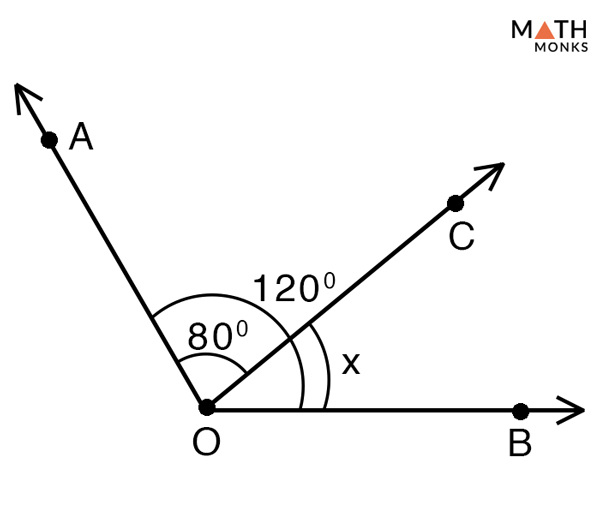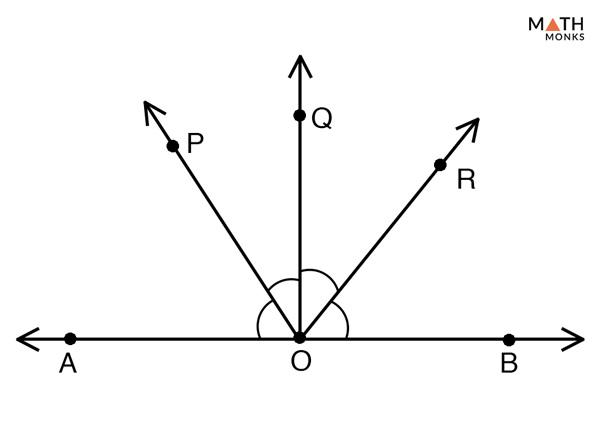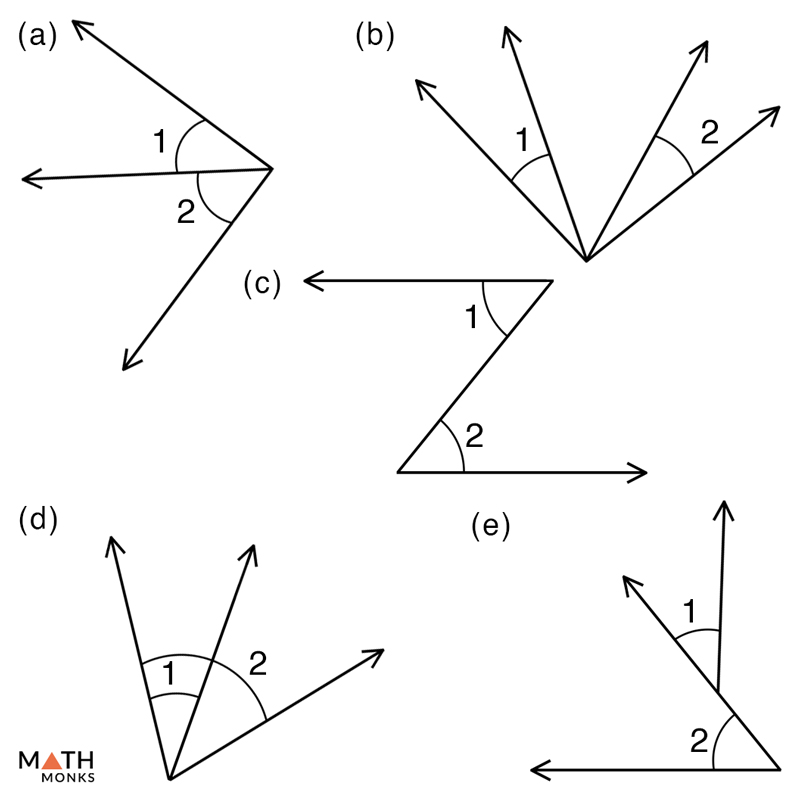### #ezw_tco-2 .ez-toc-widget-container ul.ez-toc-list li.active::before { background-color: #ededed; } chapter outline

Two angles are said to be adjacent if they share a common arm and a common vertex between them. In simple words angles that are formed side by side are called adjacent angles. One of the main features of adjacent angles is that they do not overlap. Angles that are not adjacent are called nonadjacent angles.

The figure above shows two adjacent angles having a common side and a common vertex.

### Are Adjacent Angles Complementary or Supplementary

Adjacent angles can be both complementary and supplementary. When the two angles add up to 90° it is called adjacent complementary angles, whereas when the two angles add up to 180° it is called adjacent supplementary angles. Two adjacent supplementary angles form a linear pair. See our ‘Complementary and Supplementary Angles’ article for more details.

## How to Find Adjacent Angles

To identify whether the angles are adjacent or not, we must remember its basic properties that are given below:

• They should share a common arm between them
• They should share a vertex between them
• They should not overlap
• They should have a non-common arm on both the sides of the common arm

Adjacent angles are congruent only when their common side bisects their sum. This happens when:

• A right angle is bisected to from two adjacent angles each measuring 45°
• A straight angle is bisected to from two adjacent angles where each of them is a right angle measuring 90°Solve to find the adjacent angle marked x. Given that ∠AOB = 120° and ∠AOC = 80°

Solution:

Given ∠AOB = 120° and ∠AOC = 80°
Since, ∠AOC and ∠COB are adjacent angles
Thus, ∠AOC + ∠COB = ∠AOB, here ∠COB = x
80° + x = 120°
x = 120° – 80°
x = 40°Which one of the pairs of angles given below is adjacent in the given figure.

Solution:

∠AOP and ∠POQ, ∠POQ and ∠QOR, ∠QOR and ∠ROB are three adjacent pairs of angles in the given figure.Which one of the pairs of angles given below are adjacent? Give reasons for you answer.

Solution:

a) ∠1 and ∠2 are adjacent angles because both share a common side and a common vertex
b) ∠1 and ∠2 are nonadjacent angles because they don’t share a common side
c) ∠1 and ∠2 are nonadjacent angles because they don’t have a common vertex
d) ∠1 and ∠2 are nonadjacent angles because they overlap each other
e) ∠1 and ∠2 are nonadjacent angles because they don’t share a common vertex

## Examples in Real Life

• An opened book with a page in between them
• Hands of a clock showing 3PM and the seconds hand pointing towards the digit 2
• Angles formed at the center of a cartwheel
• A sliced pizza or a cake